• 创意生成：利用素材(标题、图片、视频、落地页等)生成候选创意(用户看到的广告)
• 创意优选：从计划的候选创意(一个计划下的候选往往有多个)中选择 topk 个用于投放
• 创意投放：将优选出来的创意投放至线上

## 问题建模

online advertising 的绝大多数方法，如targeting、ranking、bidding 等，本质上都是在解决在线分配问题，即让合适的广告分发给合适用户，从而使得收入最大化（同时需要考虑生态、用户体验等），因此也可以从分配的角度去构造出一个如下简单二部图的形式，左边是 user，右边则是具体的广告(在这里为创意), 连接的边表示这个用户访问了这个广告/创意，边的权重可根据具体的业务目标决定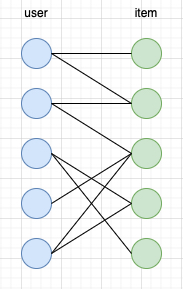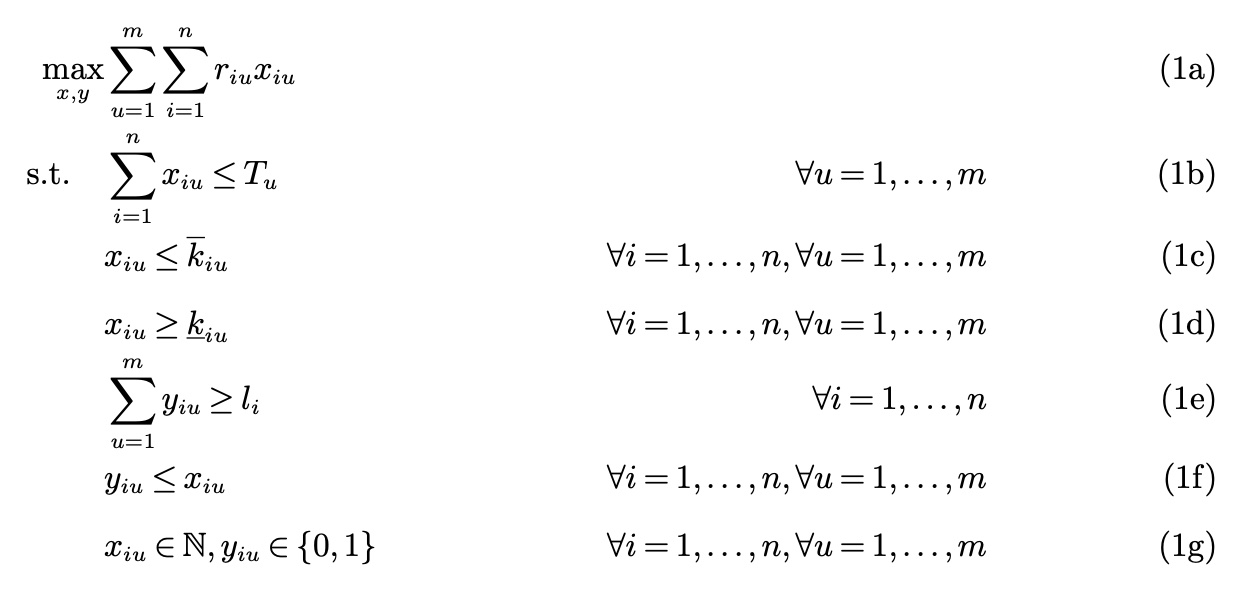• $u$: user 的 index
• $i$: item 的 index, 这里的 item 指的是创意(creative)
• $r_{iu}$: 第 $u$ 个 user 访问了第 $i$ 个 item 的 revenue，比如消耗/点击等，即上图中的边权
• $T_u$: 第 $u$ 个 user 的总访问次数
• $x_{iu}$: 第 $u$ 个 user 访问了第 $i$ 个 item 的次数
• $\underline{k_{iu}}$: 第 $u$ 个 user 访问了第 $i$ 个 item 的次数的下限
• $\overline{k_{iu}}$: 第 $u$ 个 user 访问了第 $i$ 个 item 的次数的上限
• $y_{iu}$: 第 $u$ 个 user 是否访问了第 $i$ 个 item
• $l_i$: 第 $i$ 个 item 触达的不同用户数的下限(防止只给一个用户出广告)

(1b) ad fatigue constraint, which aims to help in avoiding customers becoming fatigued by seeing a product too many times. This constraint says that a particular item $i$ can be shown at most $\overline{k_{iu}}$ times to user $u$. To calibrate ad fatigue, this parameter can be set using historical data showing when customers stop engaging with ads.
(1c) ad retargeting constraint, which focuses on showing products to customers that have seen or searched for them before. In this constraint, we say that a particular item $i$ has to be shown at least $\underline{k_{iu}}$ times to user $u$. To retarget an ad, this parameter can be set postive for items that the user saw just before the ad campaign is planned.
(1d) user diversity constraint, which addresses the requirement that advertisers want to increase reach and purchasing by showing their products to many different customers. This constraints says that at least $l_i$ users have to see a particular item $i$. These parameters are specifically set by the advertiser.

## 问题求解

### 离线求解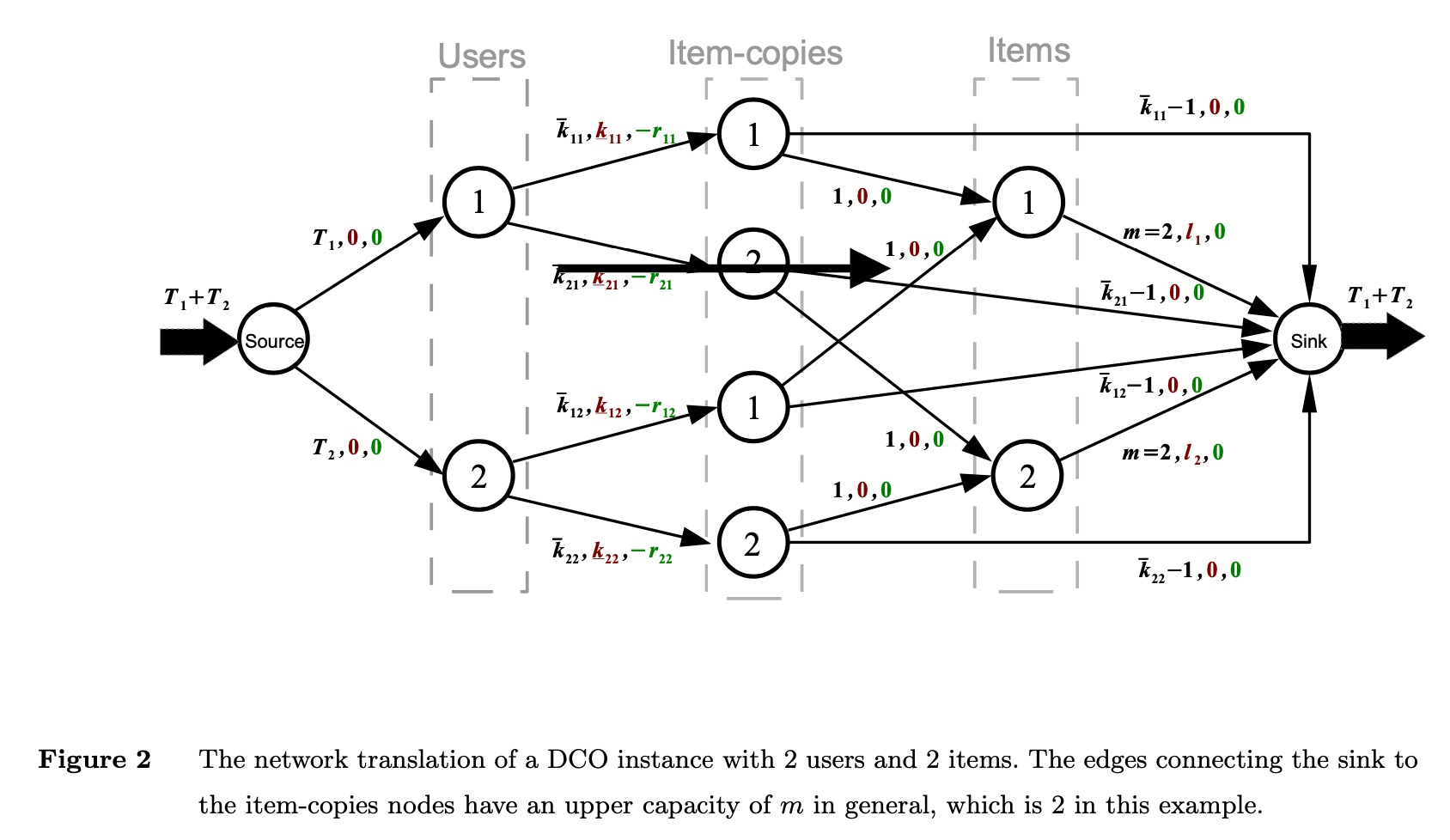• 每条边的三个参数的含义分别是 (流量上限, 流量下限, 费用)
• 从 source 出发到第 $u$ 个 user 的流量上限为 $T_u$(即用户当前访问的次数)
• 每个 user 访问每个 item 的次数的上限（ad fatigue constraint）和下限（ad retargeting constraint）的约束体现在 users->item-copies的边上
• item-copies->items 的连边是为了满足上面的user diversity constraint，即保证访问第 $i$ 个 item 的不同用户的数量不小于 $l_i$
• item->sink 中，流量上限为 $m$, 即访问第 $i$ 个 item 的不同用户数的上限是总用户数

(1)边的权值(即上面说的费用的负值)的物理含义是什么？缺失的边的权值怎么计算？
(2)采用 id 粒度过小可能导致整个图可能过大，进而导致图的求解比较困难，如果做 clustering 要怎么做？
(3)新 uid/item-id 如何处理？

### 在线求解

#### deterministic algorithm

paper 指出了 deterministic algorithm（简单来说就是不会有 random 操作，反例是启发式算法）对于在线问题表现效果很差，至于有多差，paper 给了一些证明，这里略过过程，只给出其中一些核心概念和结论

paper 在这个过程中使用了 competitive ratio来衡量 deterministic 算法的效果，这个指标主要用来描述极端情况下，online algorithm 的效果相比于 offline 的有多差，引用这个讲义里的定义如下

The competitive ratio of an online algorithm for an optimization problem is simply the approximation ratio achieved by the algorithm, that is, the worst-case ratio between the cost of the solution found by the algorithm and the cost of an optimal solution.

Proposition 1. The competitive ratio of any deterministic algorithm for the
online DCO problem is arbitrarily small

Proposition 2. Suppose that the revenue associated to all item and user pairs are bounded, that is, there exists an $r$ such that $\underline{r} ≤ r_{iu} ≤ 1$ for all items $i$ and users $u$. In this case, the competitive ratio of any deterministic algorithm for the online DCO problem is $\underline{r}$

#### deterministic algorithm with assumption

) 的一个结论，认为这个数据是比较容易能得到的，paper 描述如下，

Using historical data, advertisers can make accurate estimates about the minimum number of times they expect a user to visit the platform during the advertising period (Interactive Advertising Bureau 2020).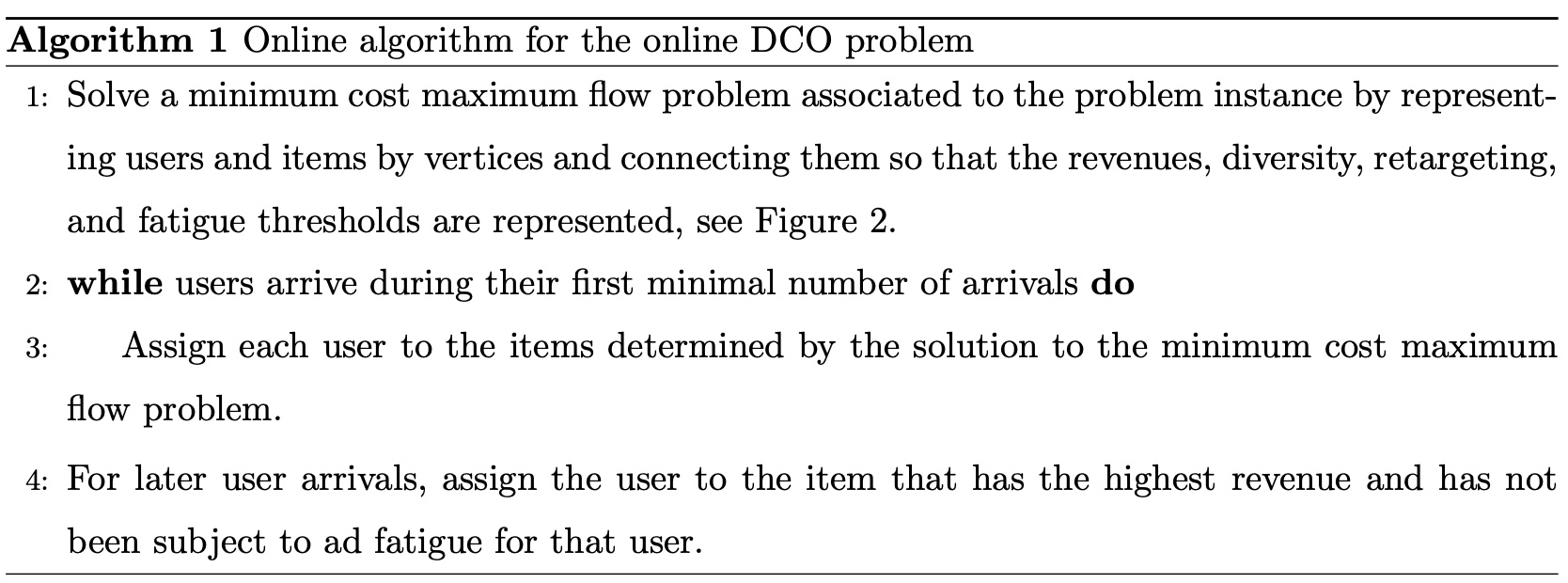paper 里绕了一大圈才给出了上面的算法流程，给出了各种符号、 lemma、proposition，但是跟最终的结论的关系又不是非常大，有点凑字数的感觉；而且比较核心的部分即 $c_u$ 是如何获取的则是基本没怎么提。。。

paper 里还给出了这个算法的 competitive ratio 的 lower bound 是（证明过程可参考 paper，这里略过）

$$1-\frac{(\overline{r} - \underline{r})n_1}{\underline{r}(n_1+n_2)}$$

• $n_1=\sum_{u=1}^{m} c_u$: 即所有用户的最少访问次数之和
• $n_2= T- n_1$: $T$ 是所有用户的实际访问次数之和
• $\underline{r}=\min_{i=1 \dots n, u=1 \dots m}r_{iu}$: 即所有 pair 的 revenue 的最小值
• $\overline{r}=\max_{i=1 \dots n, u=1 \dots m}r_{iu}$: 即所有 pair 的 revenue 的最大值

#### greedy algorithm

paper 里还提到到了另一种贪心的算法，其 assumption 是每个 item/user 都有一个reserved revenue, 第 $i$ 个 item 的 reserved revenue 记为 $\alpha_i$, 第 $u$ 个 user 的 reserved revenue 记为 $\beta_u$, 则有

$$r_{iu} = \alpha_i + \beta_u$$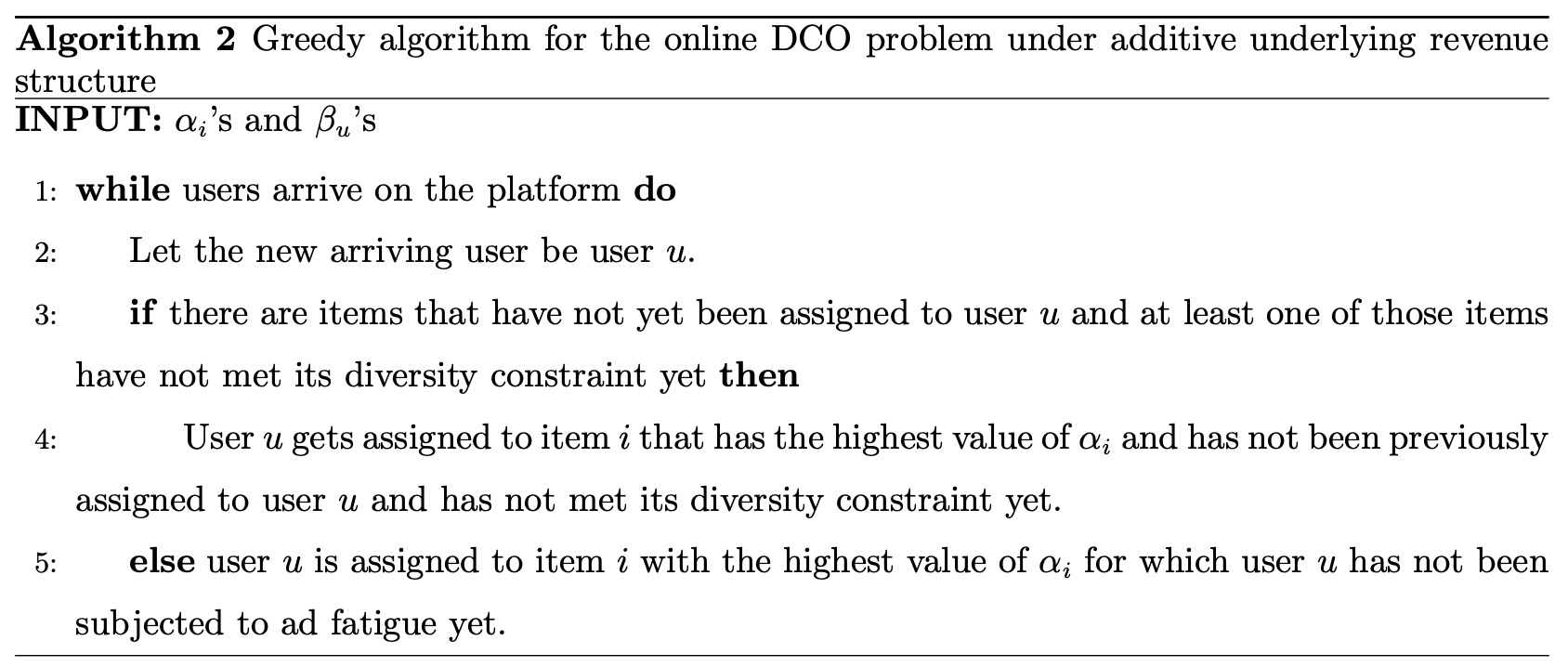## 小结

（1）如果求解出来某个 item 需要分配给某个 user，paper 里说了是直接展示，但是在实际的系统中往往是“召回+精排”的结构，需要如何保证这个 item 一定能被 user 看到？或者说，实际中的系统往往已经有一套召回和精排的策略，怎么让这套新策略融入现有的系统中

（2）user-item 边的权值的物理含义是什么？缺失的边的权值怎么计算？

（3）采用 id 粒度过小可能导致整个图可能过大，进而导致图的求解比较困难，可以怎么做，如果做 clustering 怎么保证两次聚类的结果差异性不大？

（4）新 uid/item-id 如何处理？